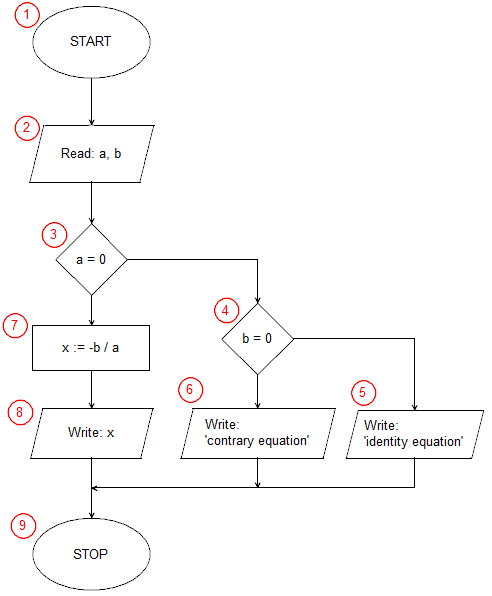# Implementation of a Linear equation solving algorithm in Pythona = float(input('a = ')) b = float(input('b = ')) if a == 0: if b == 0: print('Identity equation') else: print('Contrary equation') else: x = -b/a print('x =', x)

In the 1st line of the program, using the input() function, we read a floating-point number (of the float type) from the standard input (keyboard), which we immediately store in the variable a. The input function simultaneously displays the text given as its argument on the screen. In the 2nd line, we ask the user to enter the second number, which we assign to the variable b. Lines 1 and 2 perform input/output operations included in (2) block of the algorithm. The start block (1) has no equivalent in Python.
The conditional statement on line 3 is the selection block (3) of our algorithm. The instruction checks whether the coefficient a is equal to 0. When the condition is met, the instructions written with indentation in lines 4 to 7 are executed (in the algorithm, the selection block (4) and input-output blocks (5) and (6)). When the condition is not met, the instructions written indented after the else keyword are executed, contained in lines 9 and 10. First, in line 9 we calculate the solution to the equation x = -b/a (operation block (7)), and then in line 10 we write the result obtained (input-output block (8)).
This is where our program ends. There is no separate statement in Python that tells you when a program ends.